01 统计基础（上）：系统掌握指标的统计属性

## 指标的统计属性，指的是什么？

• 均值类的指标，比如用户的平均使用时长、平均购买金额、平均购买频率，等等。
• 概率类的指标，比如用户点击的概率（点击率）、转化的概率（转化率）、购买的概率（购买率），等等。

“随机”就代表着可以取不同的数值。比如，一款社交App每天的使用时间，对轻度用户来说可能不到1小时，而对重度用户来说可能是4、5小时以上。那么问题来了，在统计学中，怎么表征呢？

• 什么是正态分布？什么是二项分布？
• “数量足够大”具体是需要多大的数量?
• 概率类指标，为什么可以既服从二项分布又服从正态分布？

## 正态分布（Normal Distribution）

$- f(x)=\\frac{1}{\\sigma \\sqrt{2 \\pi}} e^{-\\frac{1}{2}\\left(\\frac{x-\\mu}{\\sigma}\\right)^{2}}-$

- \\begin{aligned}- \\mu &=\\frac{x\_{1}+x\_{2}+\\cdots+x\_{n}}{n} \\\\\\- \\sigma &=\\sqrt{\\frac{\\sum\_{i}^{n}\\left(x\_{i}-\\mu\\right)^{2}}{n}}- \\end{aligned}-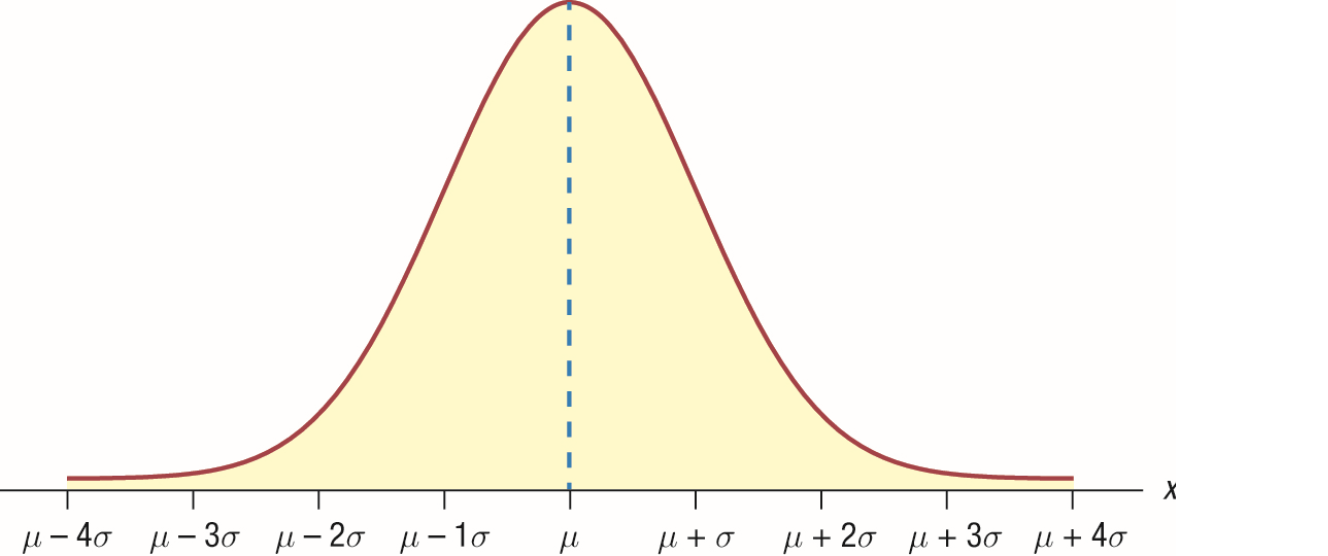from scipy.stats import norm
import numpy as np
import matplotlib.pyplot as plt

## 构建图表
fig, ax = plt.subplots()
x = np.linspace(-10,10,100)
sigma = [1.0, 2.0, 3.0, 4.0]
for s in sigma:
ax.plot(x, norm.pdf(x,scale=s), label='σ=%.1f' % s)

## 加图例
ax.set_xlabel('x')
ax.set_ylabel('Density')
ax.set_title('Normal Distribution')
ax.legend(loc='best', frameon=True)
ax.set_ylim(0,0.45)
ax.grid(True)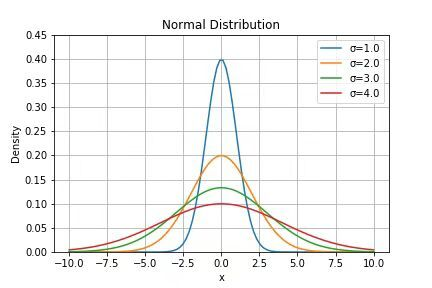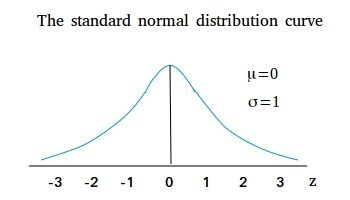$- \\mathrm{z} \\text { score }=\\frac{x-\\mu}{\\sigma}-$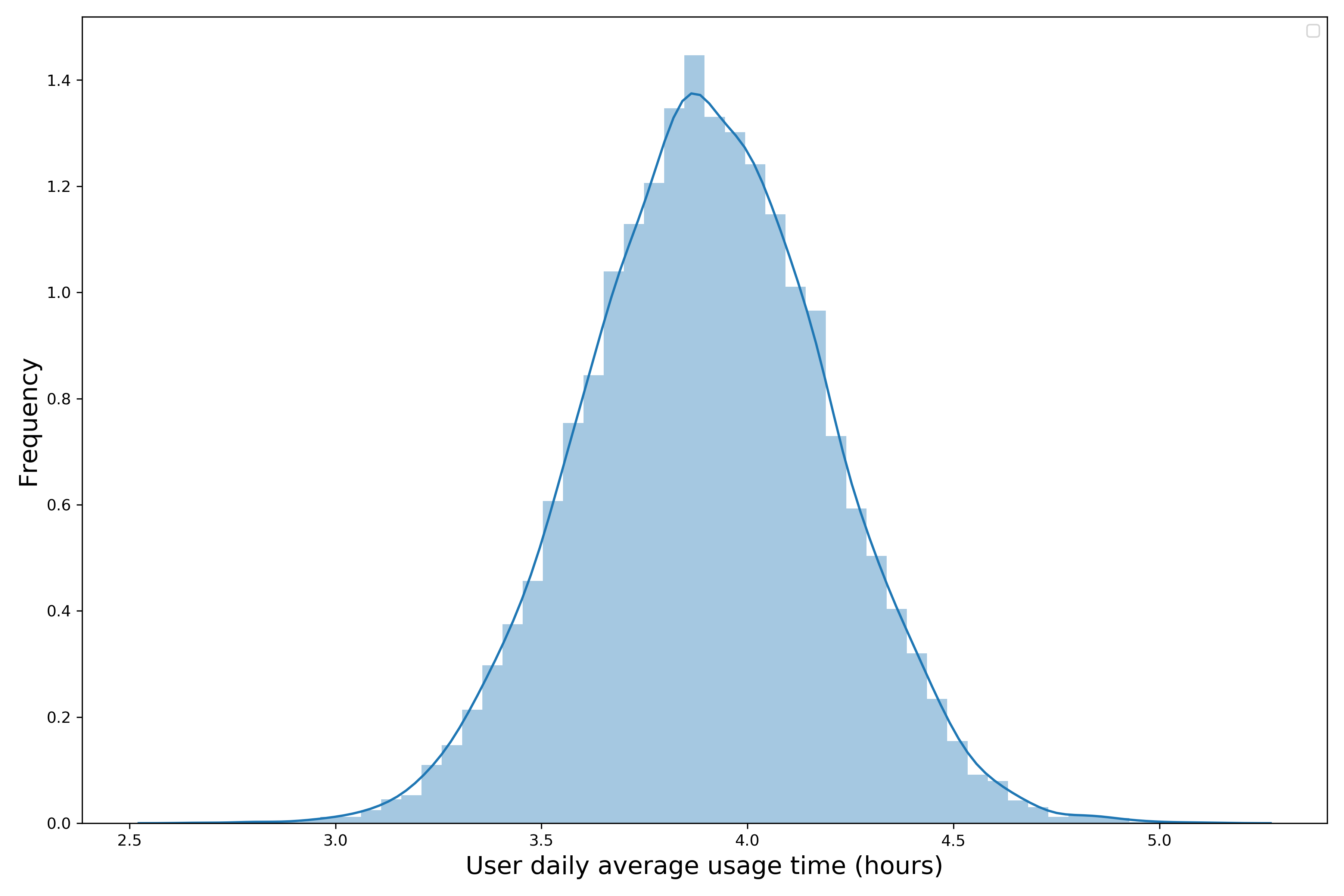## 二项分布（Binomial Distribution）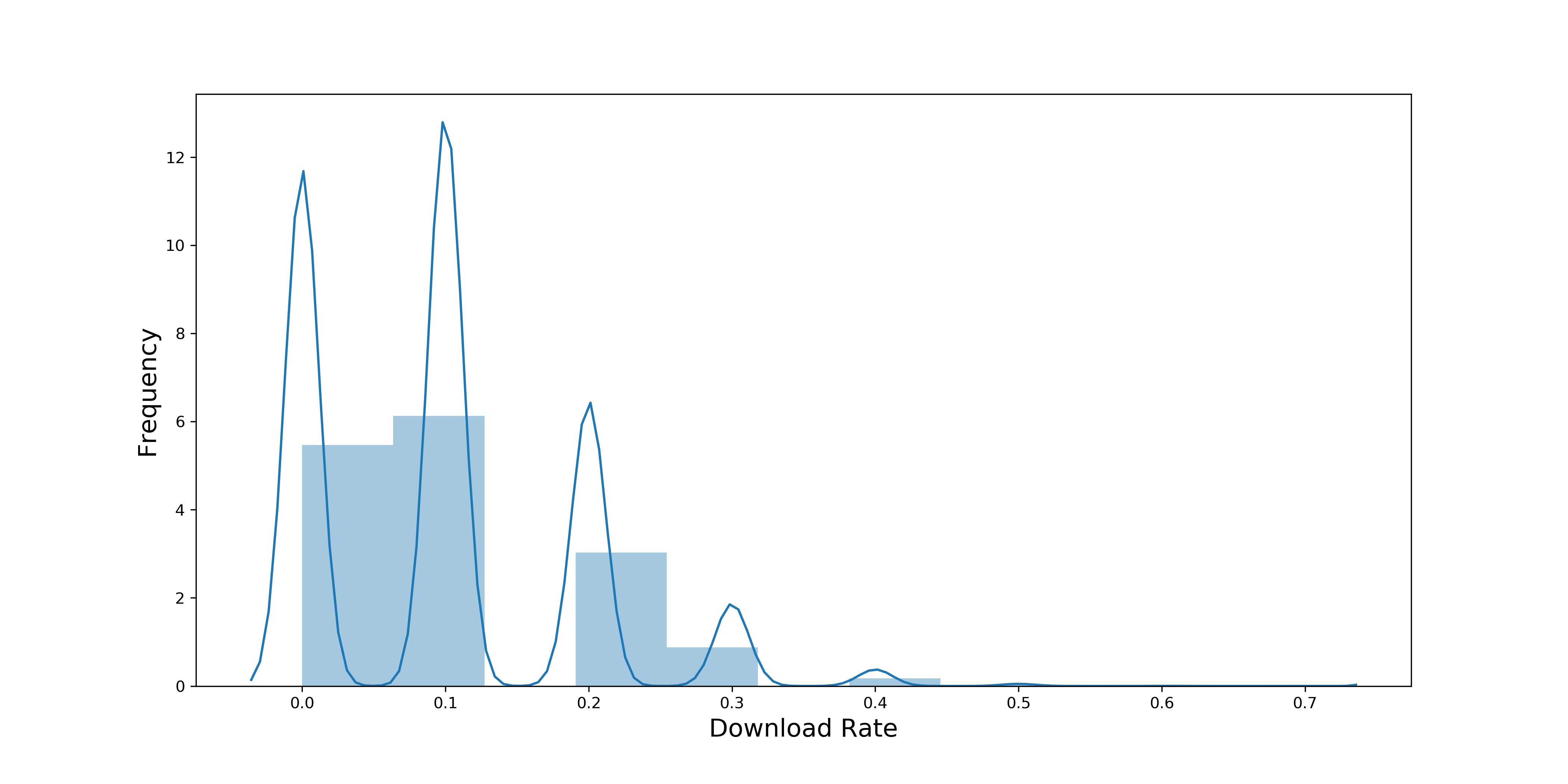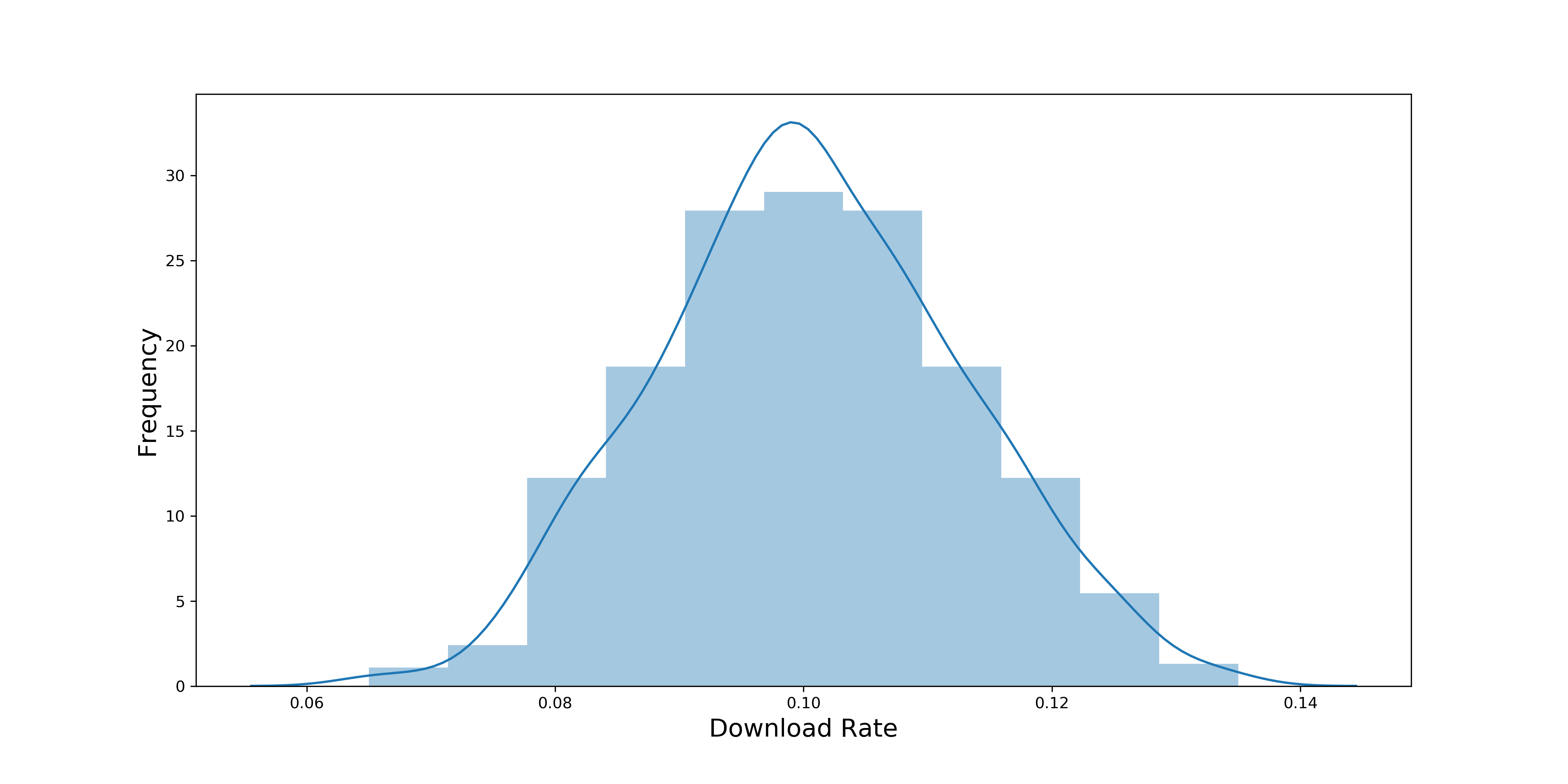## 小结

1. 一个定理：中心极限定理。
2. 两个分布：正态分布和二项分布。
3. 三个概念：方差，标准差和z分数。